# 云南烟后套种豌豆的气候特征及影响分析Analysis on Climatic Characteristics and Influence of Interplanting Pea after Growing Tobacco in Yunnan

DOI: 10.12677/CCRL.2018.73020, PDF, HTML, XML, 下载: 599  浏览: 902  国家科技经费支持

Abstract: There are 91 counties in Yunnan province where tobacco is grown, accounting for 35%~40% of the whole country in terms of planting area and purchasing quantity. Interplanting beans in tobacco fields has become an important part of Yunnan plateau characteristic planting. In order to make effective use of the late autumn climate resources with sufficient light and moderate dry heat after smoking in Yunnan tobacco-growing areas and develop the characteristic planting of interplanting rice peas after smoking, this paper takes the analysis of climate characteristics in the growing period of interplanting late autumn peas after smoking in Yaoan tobacco-growing areas in central and northern Yunnan as an example, and uses empirical orthogonality, climate tendency, Mann-Kendall mutation test and wavelet periodic analysis to analyze the comprehensive climate influence and precipitation and temperature change characteristics of climate factors such as temperature, precipitation, sunshine and freezing days in Yaoan from 1961 to 2014 ( August to December). The results show that: during the growing period of interplanting beans after smoking, light in recent 56 years and the precipitation from August to December showed a significant decreasing trend of 21.2/10a, and the trend of change was significant, with abrupt changes in 1977 and 2002 respectively. The accumulated temperature showed a significant increasing trend of 23.0/10a d, and there was a mutation in 1997. The decreasing trend of frost days is 1.1˚C/10a with the most significant change, and mutations occurred in 1964, 1966 and 1976. The average minimum air temperature in cold period showed no obvious upward trend of 0.2˚C/10a. The main oscillation periods influenced by main components of comprehensive climate impact index, dry and wet factors and heat factors are 17a, 16a and 7a respectively. The main oscillation period 29a and the sub-oscillation period 16a of the precipitation in the period are the most obvious after 2 - 4 years. Accumulated temperature main oscillation period is 7a; the average minimum air temperature in the later growth stage of pea has a main oscillation period of 11a and a sub-oscillation period of 7a. There are 19a, 3a parallel main oscillation periods and 7a sub-oscillation periods in the frost days of the critical period of pea yield formation.

1. 前言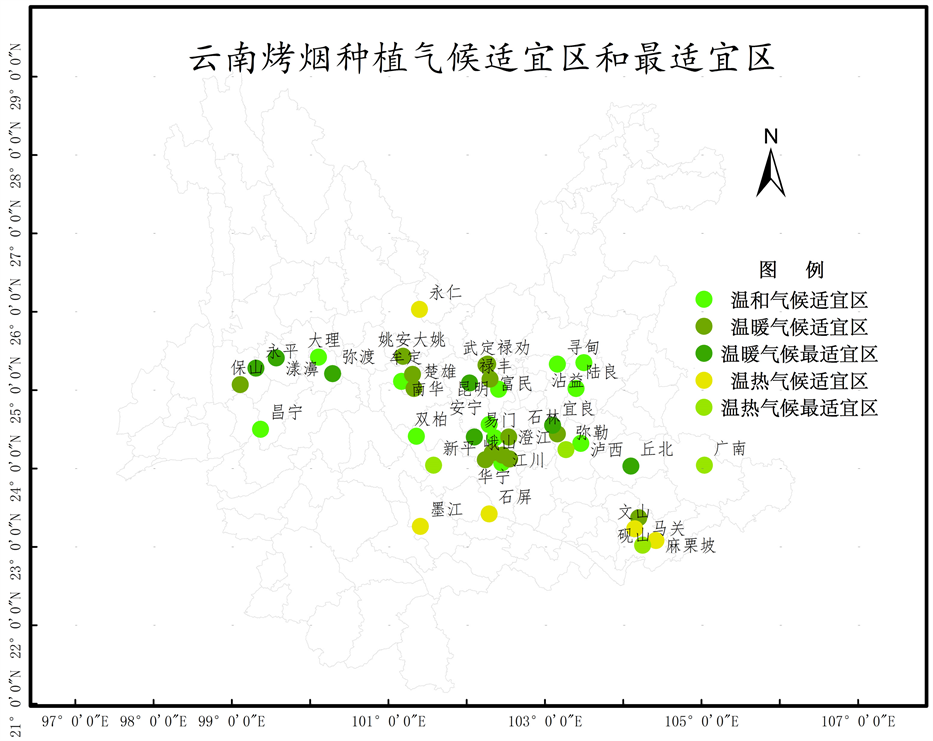Figure 1. Tobacco planting suitable climatic zones and the most suitable climate zone in Yunnan

2. 资料与方法

2.1. 资料来源

2.2. 分析时段和因子的选取

2.3. 分析方法

2.3.1. 主成分分析法的计算  - 

${Y}_{ij}=\frac{{X}_{ij}-\stackrel{¯}{{X}_{j}}}{{S}_{j}},\text{\hspace{0.17em}}\text{\hspace{0.17em}}i=1,2,\cdots ,I;\text{\hspace{0.17em}}j=1,2,\cdots ,J$

$E=\underset{k=1}{\overset{m}{\sum }}{\lambda }_{k}/\underset{j=1}{\overset{j}{\sum }}{\lambda }_{j}$

${Z}_{k}={\sum }_{j=1}^{j}{U}_{kj}{X}_{j},\text{\hspace{0.17em}}j=1,2,\cdots ,J;k=1,2,\cdots ,m$

2.3.2. 小波分析基本原理  - 

${\int }_{-\infty }^{+\infty }\psi \left(t\right)\text{d}t=\text{0}$ (1)

${\psi }_{a,b}\left(t\right)={|a|}^{-1/2}\psi \left(\frac{t-b}{a}\right)$ 其中， $a,b\in R,a\ne 0$ (2)

${W}_{f}\left(a,b\right)={|a|}^{-1/2}{\int }_{R}f\left(t\right)\stackrel{¯}{\psi }\left(\frac{t-b}{a}\right)\text{d}t$ (3)

$\Delta t$ 为取样间隔)，则式(3)的离散小波变换形式为：

${W}_{f}\left(a,b\right)={|a|}^{-1/2}\Delta t\underset{k=1}{\overset{N}{\sum }}f\left(k\Delta t\right)\stackrel{¯}{\psi }\left(\frac{k\Delta t-b}{a}\right)$ (4)

$Var\left(a\right)={\int }_{-\infty }^{\infty }{|{W}_{f}\left(a,b\right)|}^{2}\text{d}b$ (5)

2.3.3. K-M检验法(Mann-Kendall)  

${S}_{k}={\sum }_{i=1}^{k}{\sum }_{j}^{j-1}{\alpha }_{ij}\text{\hspace{0.17em}}\text{\hspace{0.17em}}\text{\hspace{0.17em}}\left(k=1,2,3,4,\cdots ,n\right)$

$U{F}_{k}=\frac{|{s}_{k}-E\left({s}_{k}\right)|}{|\sqrt{{V}_{\alpha \gamma }\left({S}_{k}\right)}|}\text{\hspace{0.17em}}\text{\hspace{0.17em}}\text{\hspace{0.17em}}\text{\hspace{0.17em}}\left(k=1,2,3,4,\cdots ,n\right)$

UFk为标准正态分布，给定显著水平а(а = 0.05, u0.05 = 1.96)，若 $|U{F}_{1}|\ge {U}_{a/2}$ ，则表明系存在明显的趋势变化。将时间系列按逆系排列，再按照上式计算，同时使

$\left\{\begin{array}{l}U{B}_{k}=-U{F}_{k}\\ k=n+1-k\end{array}\text{\hspace{0.17em}}\text{\hspace{0.17em}}\text{\hspace{0.17em}}\text{\hspace{0.17em}}\left(k=1,2,3,4,\cdots ,n\right)$

2.3.4. 气候趋势(倾向)分析方法  

${x}_{i}=a+b{t}_{i}\text{\hspace{0.17em}}\text{\hspace{0.17em}}\text{\hspace{0.17em}}\left(i=1,2,3,4,\cdots ,n\right)$

3. 结果与分析

3.1. 姚安县烤烟后套种豌豆生育期间气候特征分析

3.1.1. 综合气候影响指数的主成分(EOF)分析Table 1. Selected climate factors and codes in the studyTable 2. Xpn eigenvalues of the correlation matrix and contribution rate of varianceTable 3. Eigenvectors of the correlation matrix (Vmp matrix)

3.1.2. 时段降水量、积温和气温的气候变倾向及气候突变检验分析

3.1.3. 主成分综合气候影响指数、干湿因子分量、热量因子分量的周期变化特征

3.1.4. 烤烟后套种豌豆生育时段积温、降水、最冷时段气温和结冰日数的周期变化特征(a)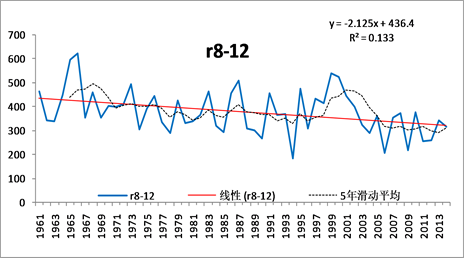(b)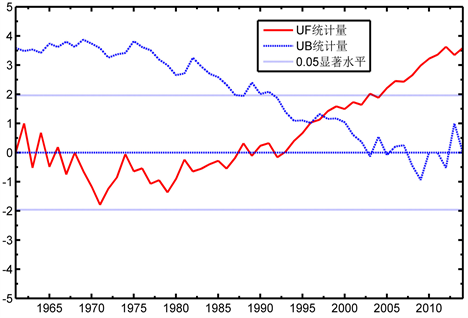(c)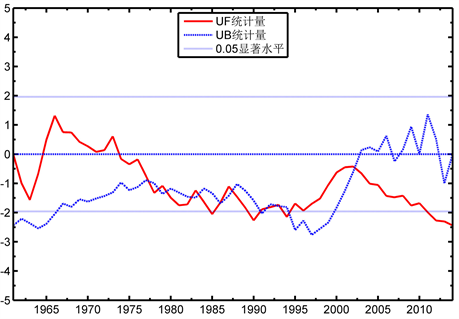(d)

Figure 2. Characteristics of precipitation and temperature and accumulated temperature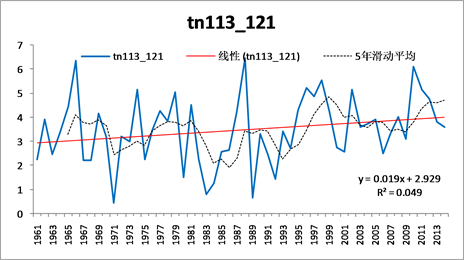(a)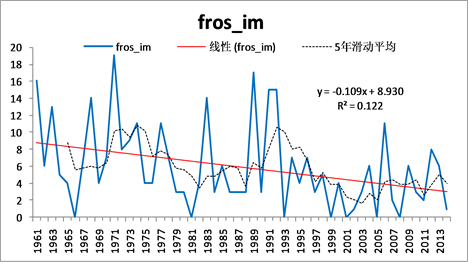(b)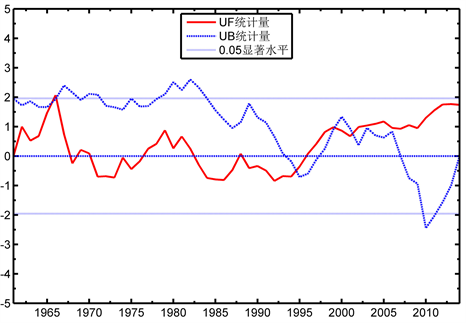(c)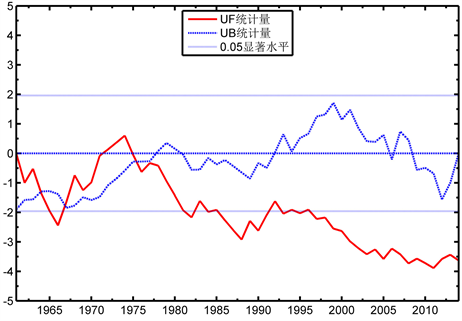(d)

Figure 3. Number of freezing days and coldest time minimum temperatures

4. 讨论与结论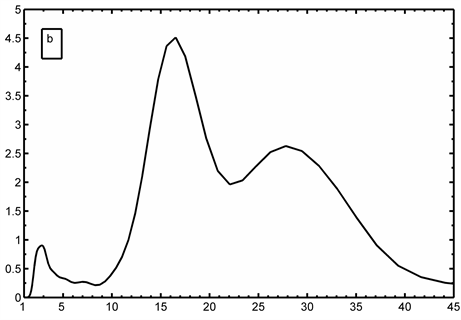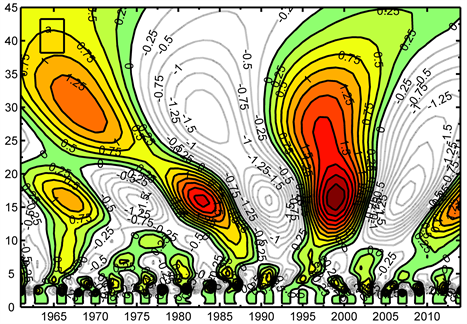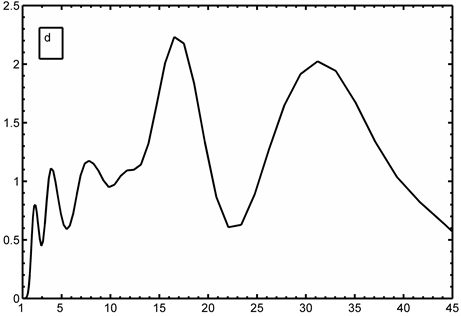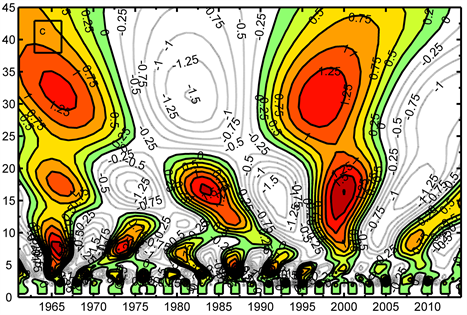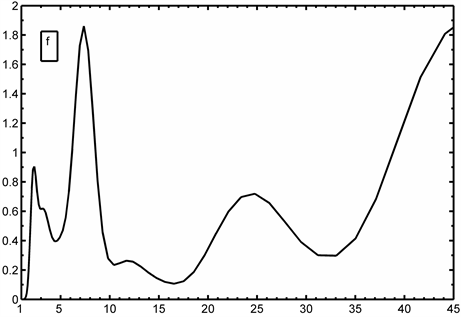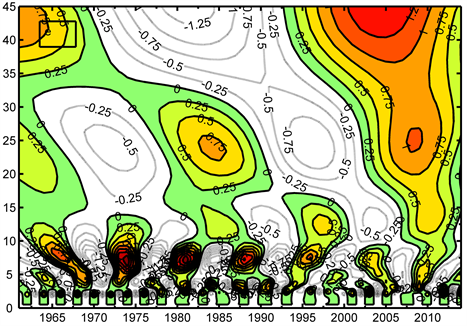Figure 4. After tobacco intercropping peas during the reproductive period (August-October), comprehensive climate index, dry and wet factor, heat cycle of wavelet and wavelet variance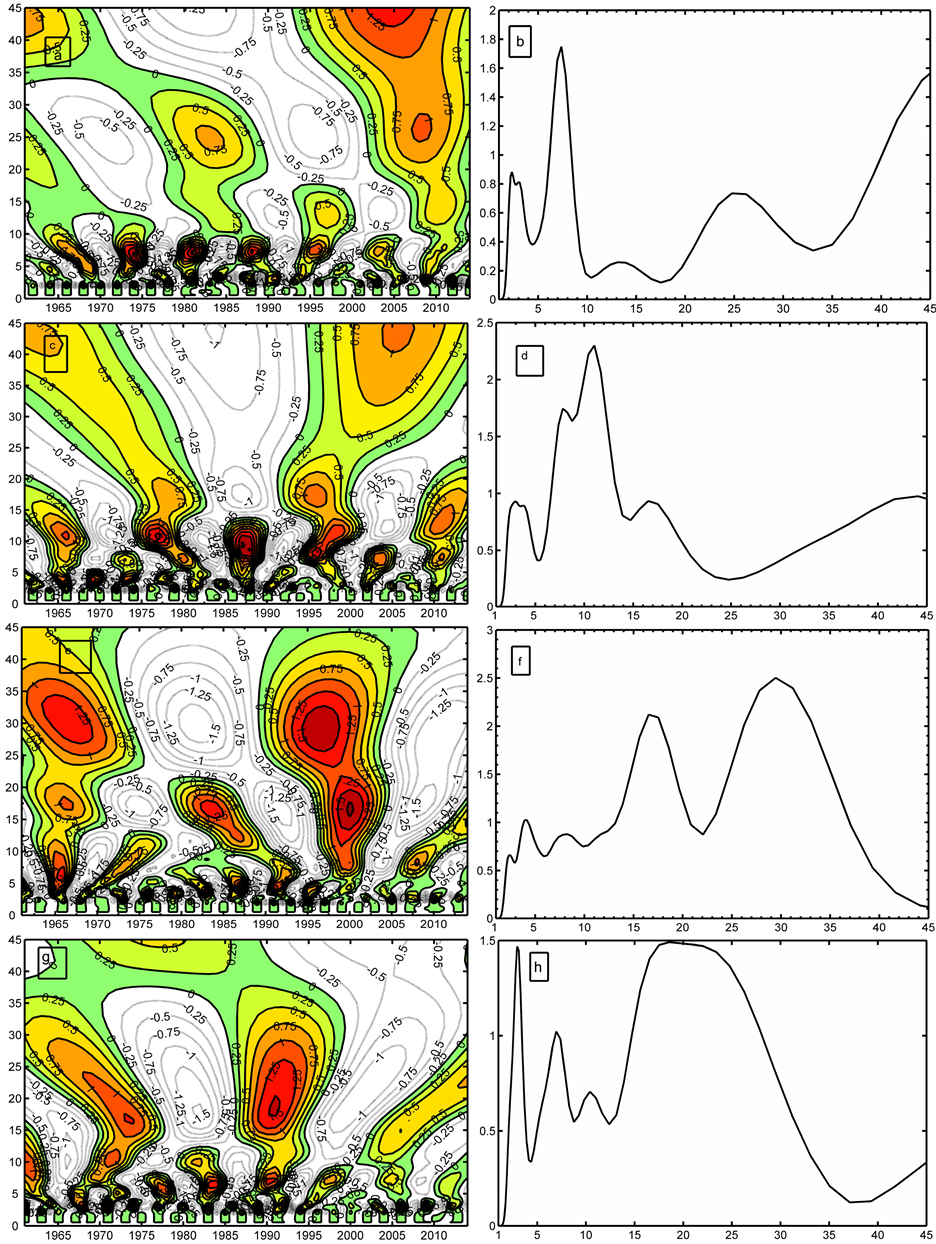Figure 5. After Tobacco intercropping peas birth-time temperature, precipitation and cold temperatures and icy day wavelet variation and wavelet variance

  邵岩, 王树会, 邓云龙, 等. 基于GIS的云南烤烟种植区划研究[M]. 北京: 科学出版社, 2009: 2-19.  胡雪琼, 黄中艳, 朱勇, 等. 云南烤烟气候类型及其适应性研究[J]. 南京气象学院学报, 2006, 29(4): 563-568.  吴景云, 杜志贵, 华云峰. 浙中地区菜碗豆翻秋栽培的气候适宜性[J]. 中国农业气象, 1997, 18(1): 30-33.  殷吉虎, 高廷科, 唐余贵, 等. 楚雄州烤烟套种主要模式及栽培技术[J]. 云南农业科技, 2009(5): 42-47.  李明福, 李洪民, 徐宁生, 等. 云南烤烟套种紫甘薯最佳栽培期试验[J]. 西南农业学报, 2013, 44(9): 1455-1458.  王鹃. 双柏县长寿仁豌豆山地栽培技术[J]. 云南农业科技, 2013(3): 43-44.  马品芬. 仁和镇烟后种植长寿仁碗豆现状及发展思考[J]. 云南农业, 2014(7): 50-51.  陈春泉. 楚雄州烤烟后种植硬壳甜脆碗豆技术[J]. 中国农技推广, 2013, 29(7): 27-28.  鲁永新, 杨永生. 楚雄州农业产业结构调整与气候[M]. 昆明: 云南民族出版社, 2004: 10-15.  彭贵芬, 刘瑜, 张一平. 云南干旱的特征及变化趋势研究[J]. 灾害学, 2009, 24(4): 40-44.  起树华, 王建彬. 元谋干热河谷气候生态环境变化的初步分析[J]. 气象研究与应用, 2007, 28(s1): 125-127.  鲁永新, 田侯明, 杨海抒, 等. 云南省野生食用菌气候生境特征与评价[J]. 中国生态农业学报, 2015, 23(6): 748-757.  穆佳, 赵俊芳, 郭建平. 近30年东北春玉米发育期对气候变化的影响[J]. 应用气象学报, 2014, 25(6): 680-689.  邢旭光, 史文娟, 张译丹, 等. 基于主成分分析法的西安市地下水资源承载力评价[J]. 水文, 2013, 33(2): 35-38.  解明恩, 张万成. 云南短期气候预测方法与模型[M]. 北京: 气象出版社, 2000: 69-81.  魏凤英. 现代气候统计诊断与预测技术[M]. 北京: 气象出版社, 2007: 106-112.  董旭光, 顾伟宗, 孟祥新, 等. 山东近50年来降水事件变化特征[J]. 地理学报, 2014, 69(5): 661-671.  彭贵芬, 刘盈曦. 云南年降水的多周期叠加与连续特大气象干旱关系及趋势预估研究[J]. 云南大学学报, 2014, 36(4): 530-537.  龙卫红, 段旭, 杞明辉. 连续小波变换在云南近百年气温和降水变化分析中的应用[J]. 高原气象, 1999, 18(1): 1-9.  张代青, 梅亚东, 杨娜, 等. 中国大陆近54年降水量变化规律的小波分析[J]. 武汉大学学报, 2010, 43(3): 278-287.  曹洁萍, 迟道才, 武立强, 等. Mann-Kendall检验方法在降水趋势分析中的应用研究[J]. 农业科技与装备, 2008(5): 35-40.  简虹, 骆云中, 谢德体. 基于Mann-Kendall法和小波分析的降水变化特征研究[J]. 西南师范大学学报, 2011, 36(4): 217-222.  田海峰, 秦耀辰, 李国栋, 等. 基于小波和M-K的豫东农区近60年气温变化的多尺度分析[J]. 中国农学通报, 2013, 29(35): 329-338.  鲁永新, 杨永生. 楚雄州气候变化与气象灾害[M]. 昆明: 云南科技出版社, 2010: 86-87.  陶云, 樊风, 段旭, 等. 云南不同气候带气温变化特征[J]. 云南大学学报, 2013, 35(5): 652-660.  王宇. 云南山地气候[M]. 云南科技出版社, 2006: 14-48.  赫维人, 陈永生, 杨明, 等. 云南农业地理[M]. 昆明: 云南人民出版社, 1981: 86-94.